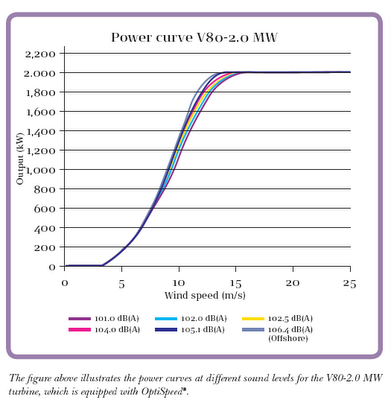# Wind Turbine Efficiency

At an NCRES meeting last night the question of wind turbine efficiency came up and I was about to explain it based on my understanding of the Betz limit, but realized it was a bit too complex an issue to summarize in a few sentences, so I decided to put it in a blog article. In fact, it’s even a little challenging to put in the blog, because it requires a table and the blog composing interface doesn’t seem to properly display tables or the characters pi or rho for some reason. So you can find a table and a few more details here, if you’re curious.

I use both the units of mph and m/s for speed since the U.S. has never bothered to convert to the metric system. For U.S. readers, an easy trick to convert from m/s to mph is to double the number and add 10%. What follows is rather technical, so if you’re not interested in math, physics, or wind energy, you may just wish to skip this article.

In order to understand how much power you can generate with a wind turbine, you must first know how much energy is available in the wind. This energy is primarily determined by wind speed and the size of the wind turbine’s rotor. Power generated is proportional to how much kinetic energy can be extracted per unit time.

Kinetic energy of a moving mass is defined by the equation ½ mv2. We need to know the kinetic energy of the air moving across the swept area of the turbine’s rotor. Multiplying energy by its rate of movement will provide its power.

The air’s mass per unit time can be computed with the formula pAv where:

p = density of the air
A = swept area of turbine’s rotor
v = velocity of the wind

Thus combining the equations for kinetic energy and wind speed, the power available in wind comes out to:

½ pAv3

Air density (p) is about 1.2 kg/m3 at sea level and a temperature of 20 °C. This number varies depending on temperature and altitude. For example, in Colorado air density is about 1 kg/m3 or about 20% less than at sea level.

Let’s take the example of a Vestas V80 turbine with an 80 meter rotor. The amount of wind energy available in a 20 mph (9.8 m/s) wind for this turbine with 5027 m2 of swept area is:

½ × 1.2 kg/m3 × 3.14 × (40 m)2 × (8.9 m/s)3

= 2.3 × 106 kg·m2/s3 = 2.3 MW

However, this number is the theoretical power of all the wind moving across the swept area, and you cannot completely stop the wind to get 100% of its energy. In 1920, a German physicist named Albert Betz figured out that the maximum energy that can be extracted by a wind turbine is about 59.3% of the theoretical energy present in the wind. This has become known as Betz’s Law. This means that you can only get about 1.35 MW from a 20 mph wind at sea level in the example mentioned above in the best case. Looking up the specifications for the Vestas V80 wind turbine in the graph below, we see that it generates about 950 kW in a 20 mph wind, which means it achieves about 70% of its Betz limit efficiency. This number is impressive considering there are losses at every stage of energy conversion, including the drag on the blades, the gearbox, the generator, and the transformer and losing only 30% through all these stages is quite good.As the wind speed increases, the energy present in it goes up by the cube of its velocity. However, the maximum output of the V80 turbine stops at about 28 mph (12.5 m/s) when it reaches 2 MW. At this point, this turbine generates at about 54% of its Betz limit efficiency. From this point on, the wind turbine will not generate more than its 2 MW rated power despite the fact that the Betz limit power will climb to nearly 30 MW by the time the cutoff of the turbine is reached at 56 mph (25 m/s). Around the time of the cutoff, therefore, the turbine will be operating at 7% of its Betz limit. The turbine cuts off in high winds to protect itself from damage.

In the grand scheme of things, much of this ‘wasted’ power is mostly hypothetical since the wind speeds even at some of the best sited wind farms tend to average between 15 to 26 mph throughout the year. If the components were to be sized to handle 30 MW of power, they would be much heavier and much more expensive. So sizing the turbine for 2MW optimizes overall cost considerations over the life of the turbine.

So, how efficient is a wind turbine? The answer is that ‘it depends on how you define efficiency’. In the case where you measure efficiency as the amount of energy that is theoretically available to what is actually extracted, it can look like a very small number, about 40% maximum. However, if you consider that without the wind turbine, 100% of the energy it provides would be wasted, then the answer is that it is infinitely efficient.

This entry was posted in energy, wind by Lee Devlin. Bookmark the permalink.1.A. Benway on said: